# Arrays II Review of arrays u Arrays are

• Slides: 38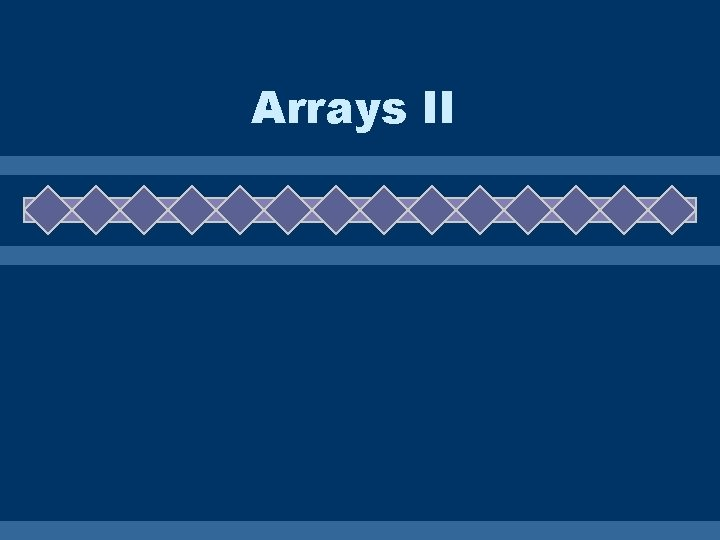Arrays II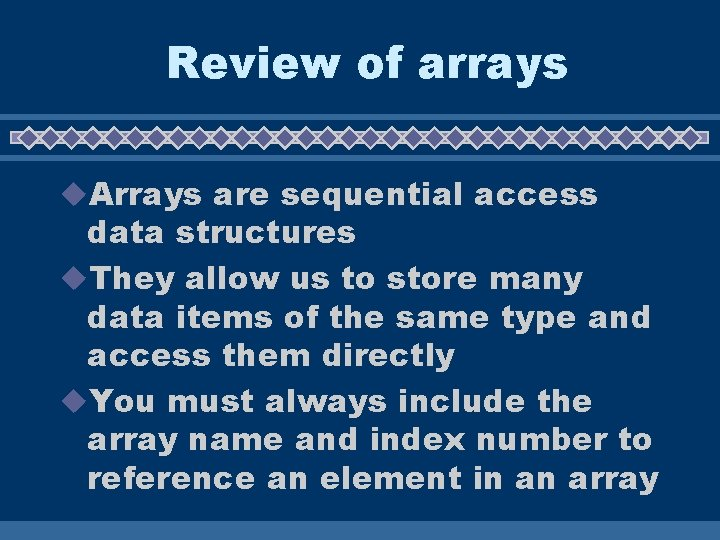Review of arrays u. Arrays are sequential access data structures u. They allow us to store many data items of the same type and access them directly u. You must always include the array name and index number to reference an element in an array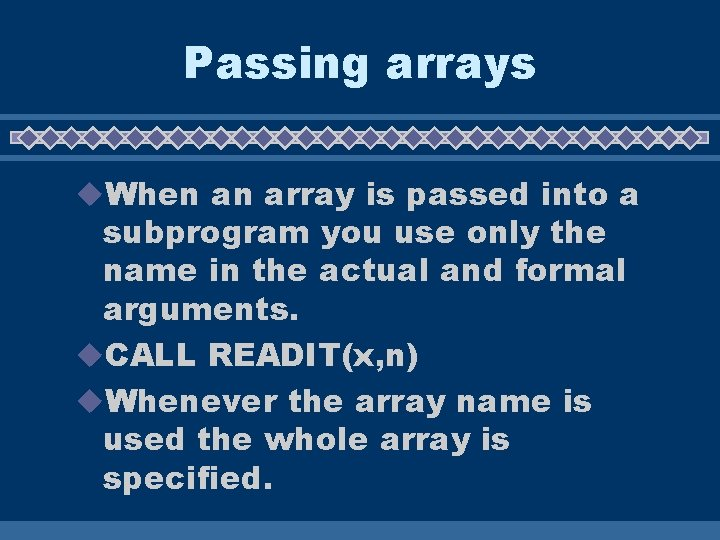Passing arrays u. When an array is passed into a subprogram you use only the name in the actual and formal arguments. u. CALL READIT(x, n) u. Whenever the array name is used the whole array is specified.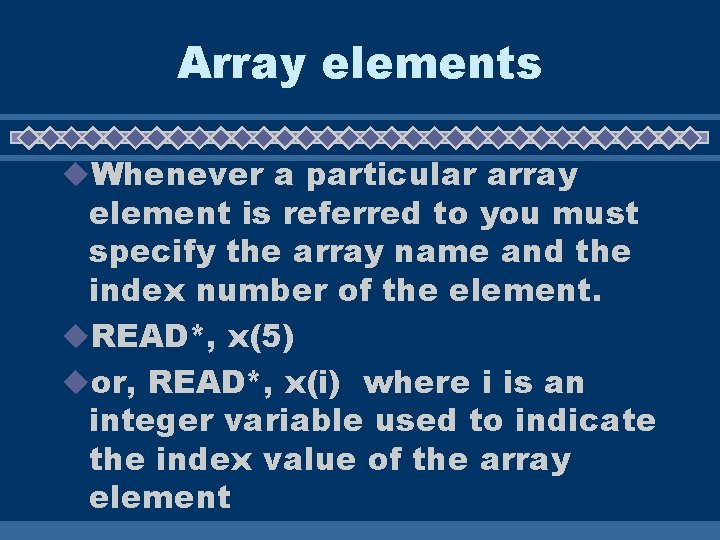Array elements u. Whenever a particular array element is referred to you must specify the array name and the index number of the element. u. READ*, x(5) uor, READ*, x(i) where i is an integer variable used to indicate the index value of the array elementCommon mistakes u. Here are two common mistakes u 1. Passing a particular element when you intent to pass the entire array – CALL READIN(x(i), n) WRONG! Should be x u 2. Referring to the entire array when you only want one element – READ*, x WRONG! Should be x(i)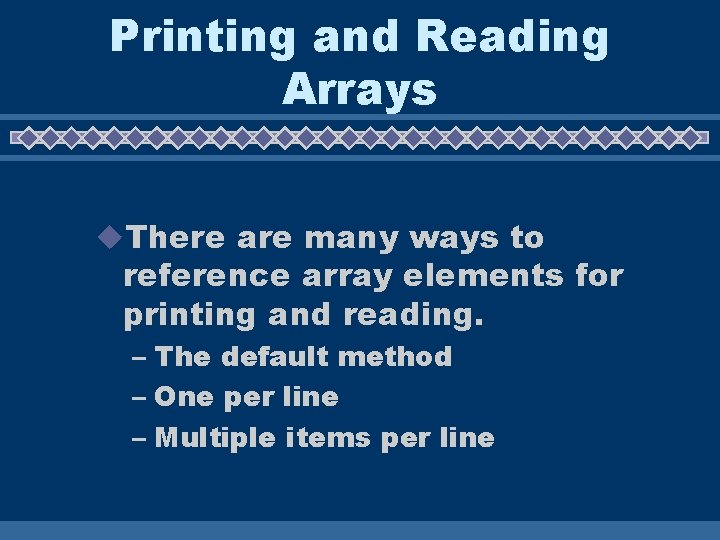Printing and Reading Arrays u. There are many ways to reference array elements for printing and reading. – The default method – One per line – Multiple items per line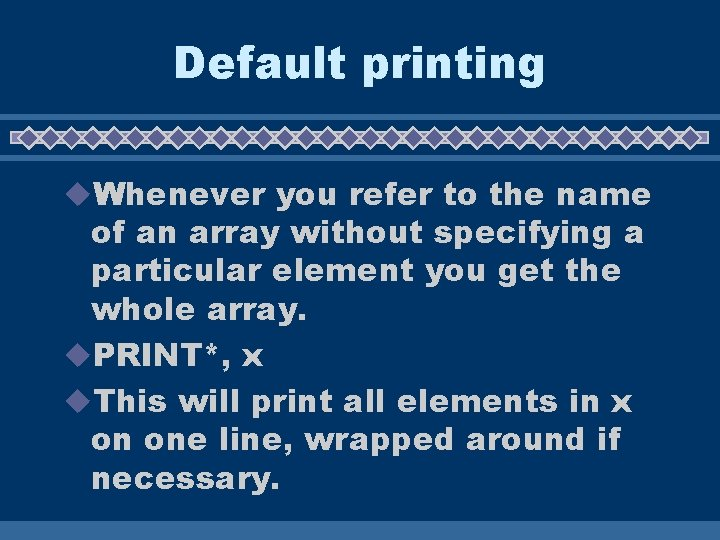Default printing u. Whenever you refer to the name of an array without specifying a particular element you get the whole array. u. PRINT*, x u. This will print all elements in x on one line, wrapped around if necessary.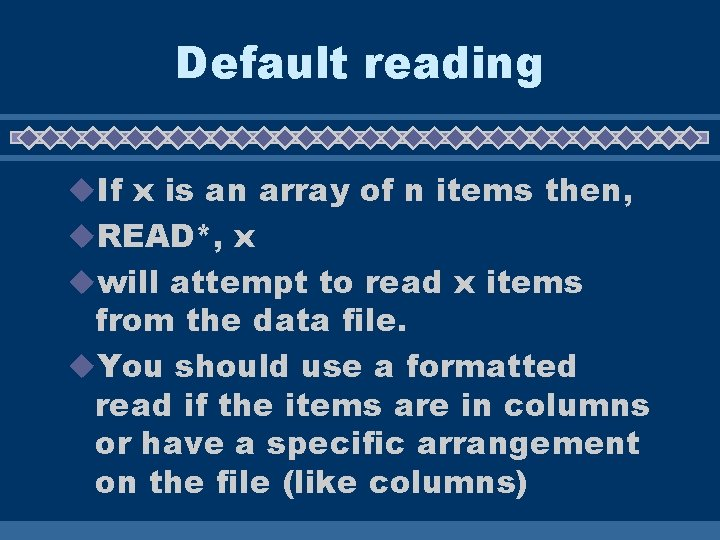Default reading u. If x is an array of n items then, u. READ*, x uwill attempt to read x items from the data file. u. You should use a formatted read if the items are in columns or have a specific arrangement on the file (like columns)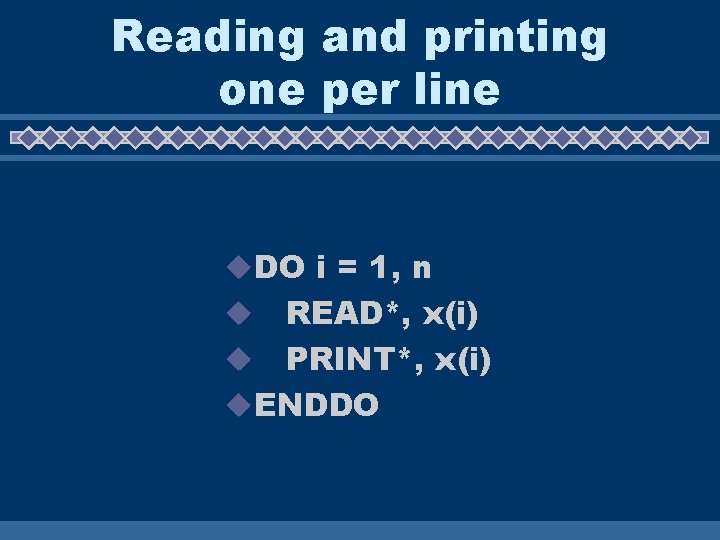Reading and printing one per line u. DO i = 1, n READ*, x(i) u PRINT*, x(i) u. ENDDO u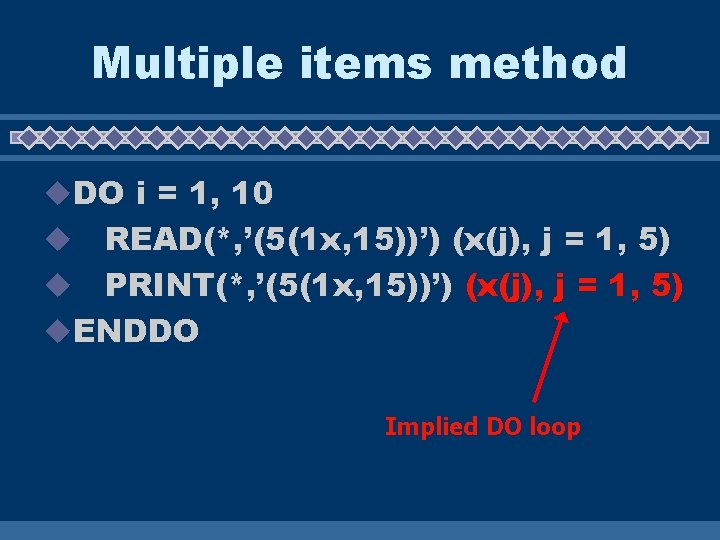Multiple items method u. DO i = 1, 10 READ(*, ’(5(1 x, 15))’) (x(j), j = 1, 5) u PRINT(*, ’(5(1 x, 15))’) (x(j), j = 1, 5) u. ENDDO u Implied DO loop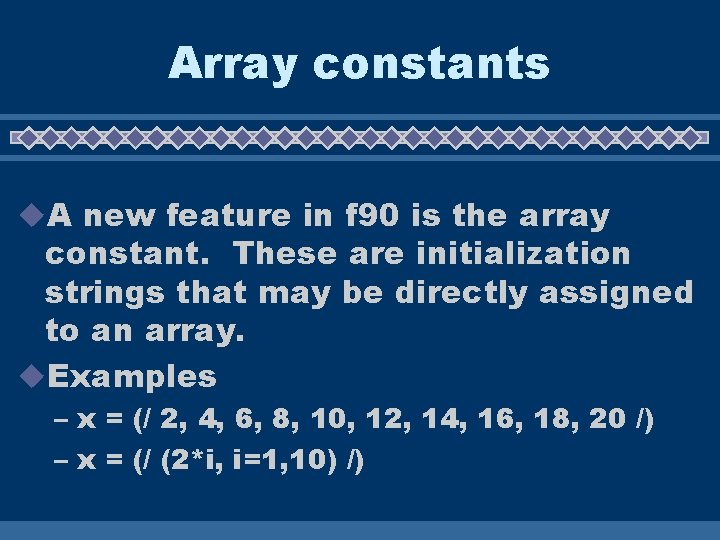Array constants u. A new feature in f 90 is the array constant. These are initialization strings that may be directly assigned to an array. u. Examples – x = (/ 2, 4, 6, 8, 10, 12, 14, 16, 18, 20 /) – x = (/ (2*i, i=1, 10) /)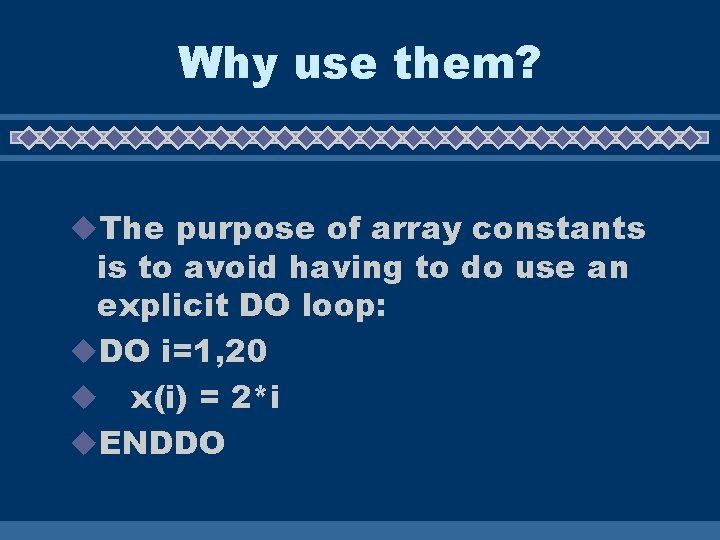Why use them? u. The purpose of array constants is to avoid having to do use an explicit DO loop: u. DO i=1, 20 u x(i) = 2*i u. ENDDO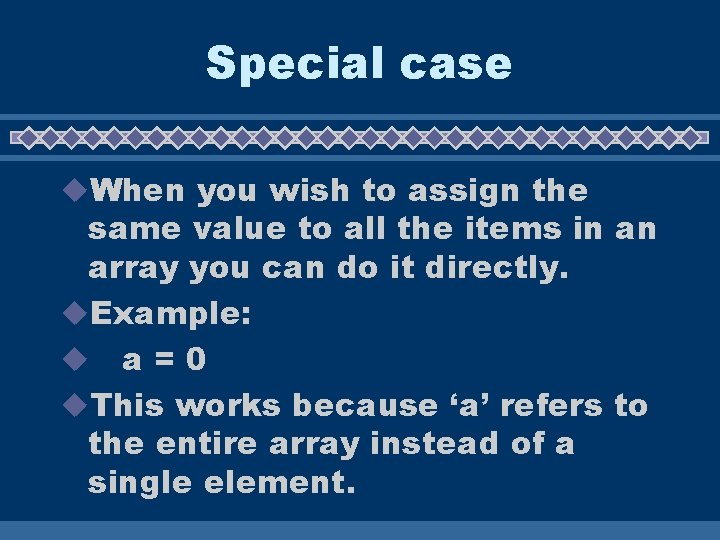Special case u. When you wish to assign the same value to all the items in an array you can do it directly. u. Example: u a=0 u. This works because ‘a’ refers to the entire array instead of a single element.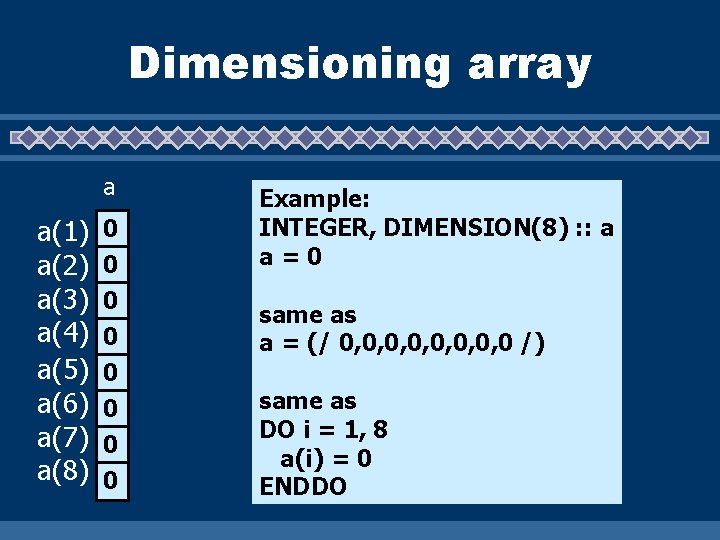Dimensioning array a a(1) a(2) a(3) a(4) a(5) a(6) a(7) a(8) 0 0 0 0 Example: INTEGER, DIMENSION(8) : : a a=0 same as a = (/ 0, 0, 0 /) same as DO i = 1, 8 a(i) = 0 ENDDO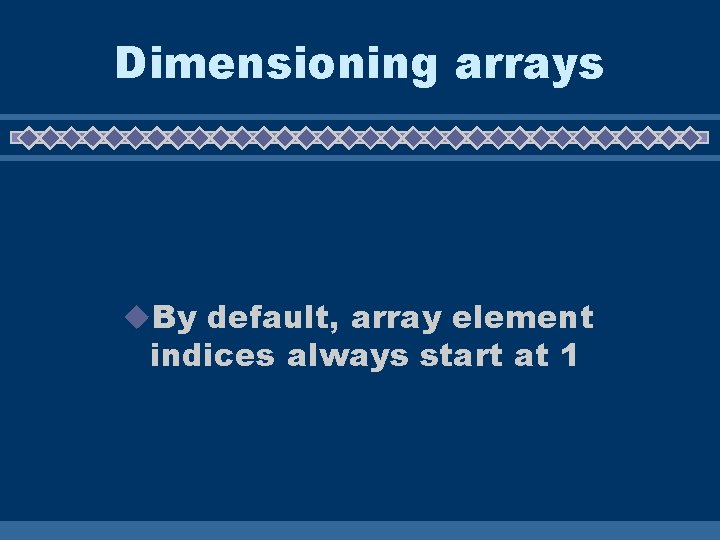Dimensioning arrays u. By default, array element indices always start at 1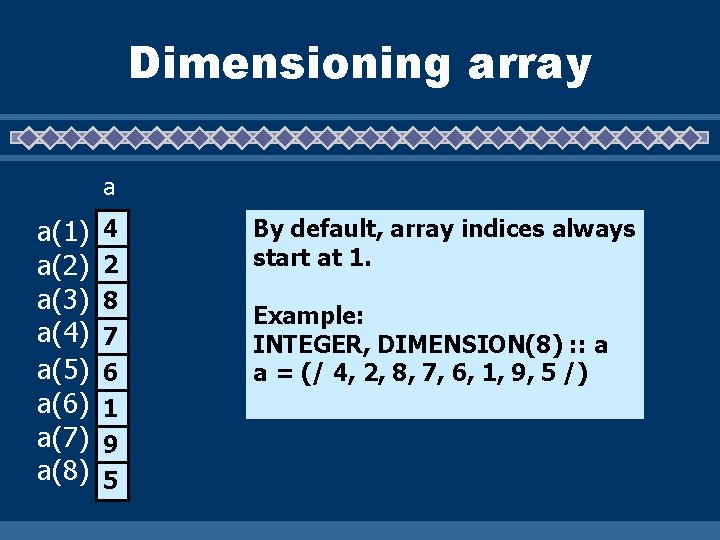Dimensioning array a a(1) a(2) a(3) a(4) a(5) a(6) a(7) a(8) 4 2 8 7 6 1 9 5 By default, array indices always start at 1. Example: INTEGER, DIMENSION(8) : : a a = (/ 4, 2, 8, 7, 6, 1, 9, 5 /)Dimensioning array a a(0) a(1) a(2) a(3) a(4) a(5) a(6) a(7) 4 2 8 7 6 1 9 5 Sometimes it is not appropriate to start at 1, if so, you can set both dimensions when the array is declared. Example: INTEGER, DIMENSION(0: 7) : : a a = (/ 4, 2, 8, 7, 6, 1, 9, 5 /)Dimensioning array a a(-3) 4 a(-2) 2 a(-1) 8 a(0) 7 a(1) 6 a(2) 1 a(3) 9 a(4) 5 Example: INTEGER, DIMENSION(-3: 4) : : a a = (/ 4, 2, 8, 7, 6, 1, 9, 5 /)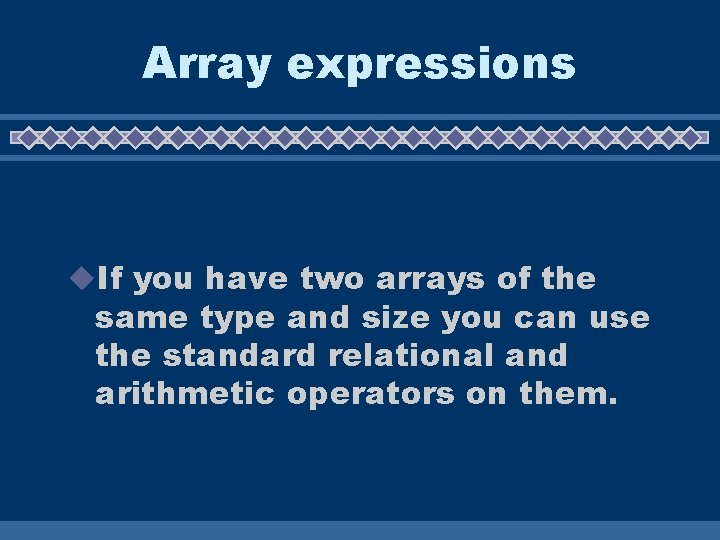Array expressions u. If you have two arrays of the same type and size you can use the standard relational and arithmetic operators on them.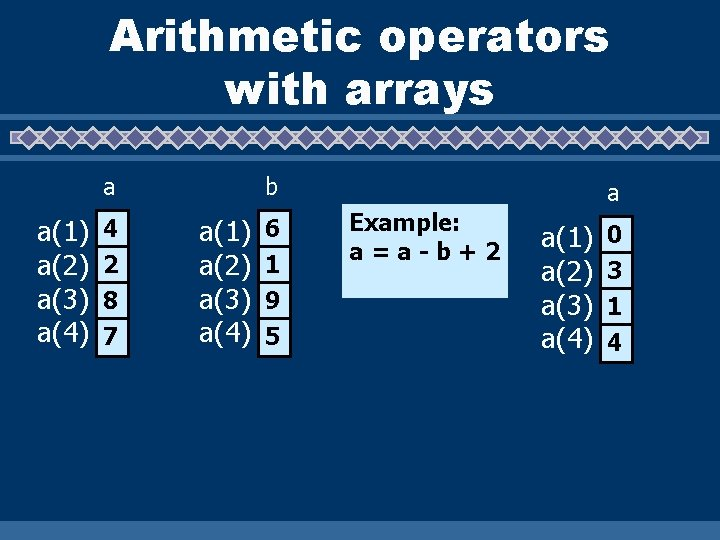Arithmetic operators with arrays a a(1) a(2) a(3) a(4) 4 2 8 7 b a(1) a(2) a(3) a(4) 6 1 9 5 a Example: a=a-b+2 a(1) a(2) a(3) a(4) 0 3 1 4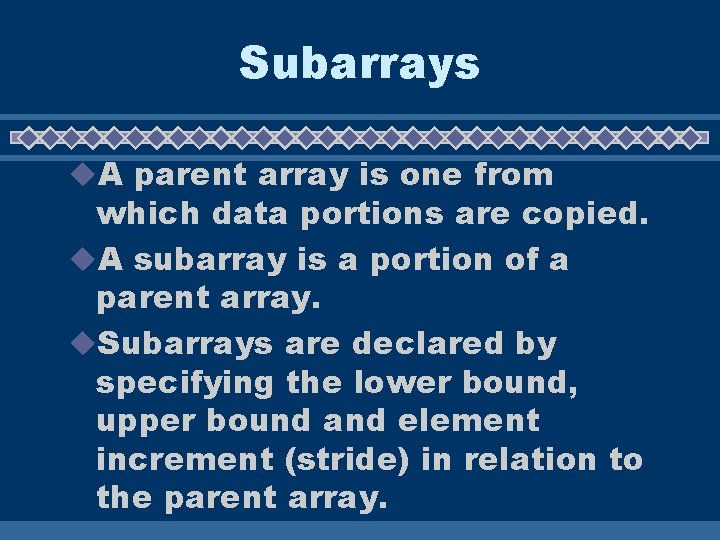Subarrays u. A parent array is one from which data portions are copied. u. A subarray is a portion of a parent array. u. Subarrays are declared by specifying the lower bound, upper bound and element increment (stride) in relation to the parent array.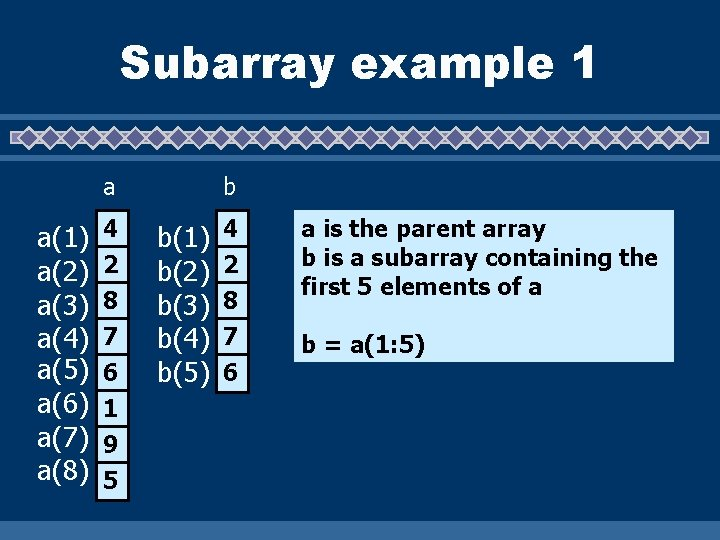Subarray example 1 a a(1) a(2) a(3) a(4) a(5) a(6) a(7) a(8) 4 2 8 7 6 1 9 5 b b(1) b(2) b(3) b(4) b(5) 4 2 8 7 6 a is the parent array b is a subarray containing the first 5 elements of a b = a(1: 5)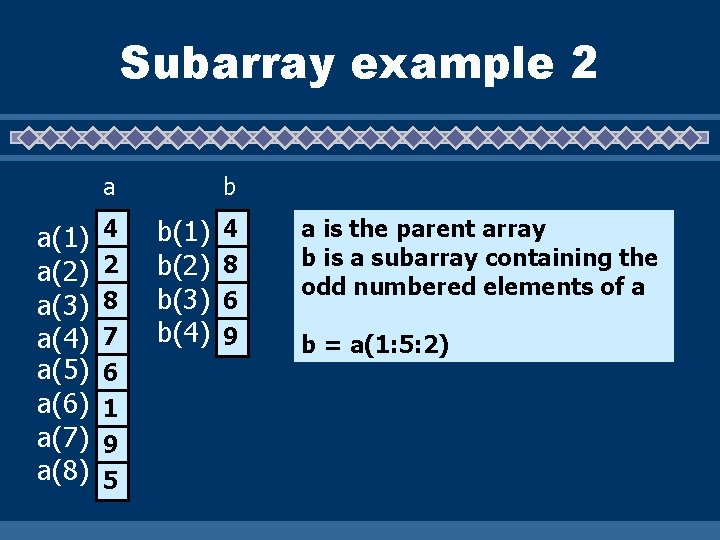Subarray example 2 a a(1) a(2) a(3) a(4) a(5) a(6) a(7) a(8) 4 2 8 7 6 1 9 5 b b(1) b(2) b(3) b(4) 4 8 6 9 a is the parent array b is a subarray containing the odd numbered elements of a b = a(1: 5: 2)Vector subscripts a a(1) a(2) a(3) a(4) a(5) a(6) a(7) a(8) 4 2 8 7 6 1 9 5 b i i(1) i(2) i(3) i(4) 1 4 5 6 b(1) b(2) b(3) b(4) 0 0 Arrays are often called vectors. Here is an example that uses an array (called a vector array) to serve as a map for the definition of parent array elements to be included in a subarray.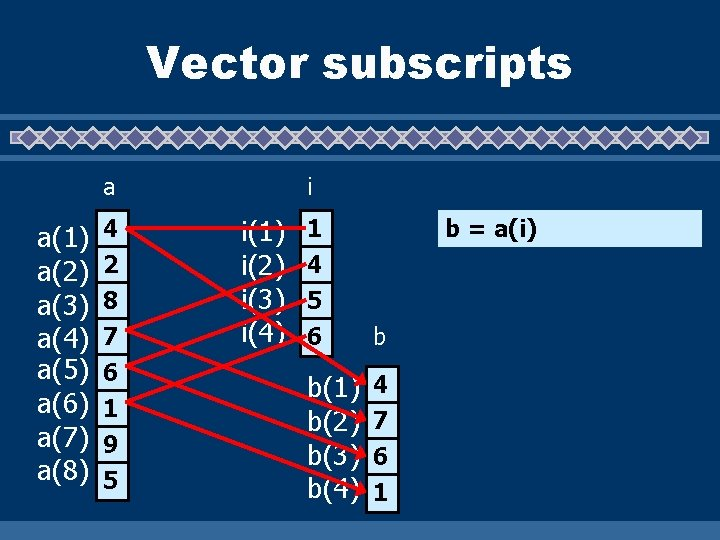Vector subscripts a a(1) a(2) a(3) a(4) a(5) a(6) a(7) a(8) 4 2 8 7 6 1 9 5 i i(1) i(2) i(3) i(4) 1 4 5 6 b = a(i) b b(1) b(2) b(3) b(4) 4 7 6 1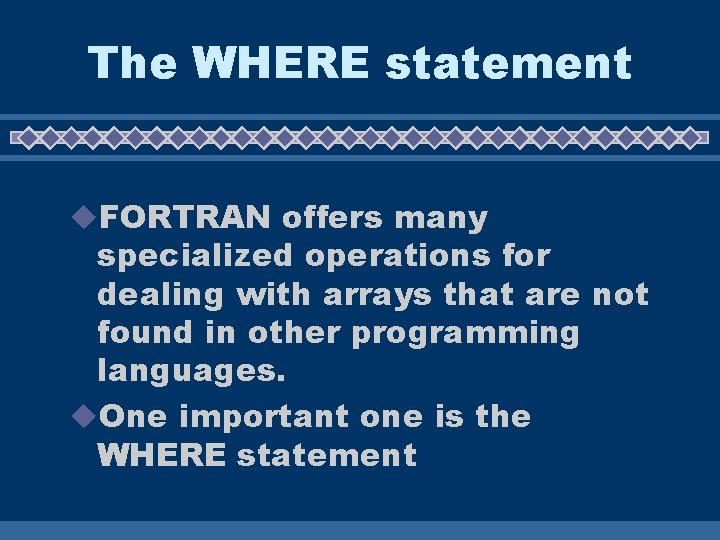The WHERE statement u. FORTRAN offers many specialized operations for dealing with arrays that are not found in other programming languages. u. One important one is the WHERE statement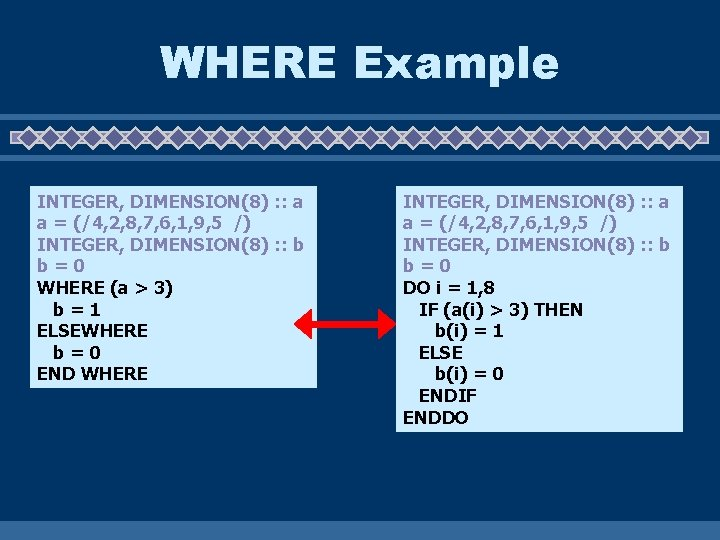WHERE Example INTEGER, DIMENSION(8) : : a a = (/4, 2, 8, 7, 6, 1, 9, 5 /) INTEGER, DIMENSION(8) : : b b=0 WHERE (a > 3) b=1 ELSEWHERE b=0 END WHERE INTEGER, DIMENSION(8) : : a a = (/4, 2, 8, 7, 6, 1, 9, 5 /) INTEGER, DIMENSION(8) : : b b=0 DO i = 1, 8 IF (a(i) > 3) THEN b(i) = 1 ELSE b(i) = 0 ENDIF ENDDO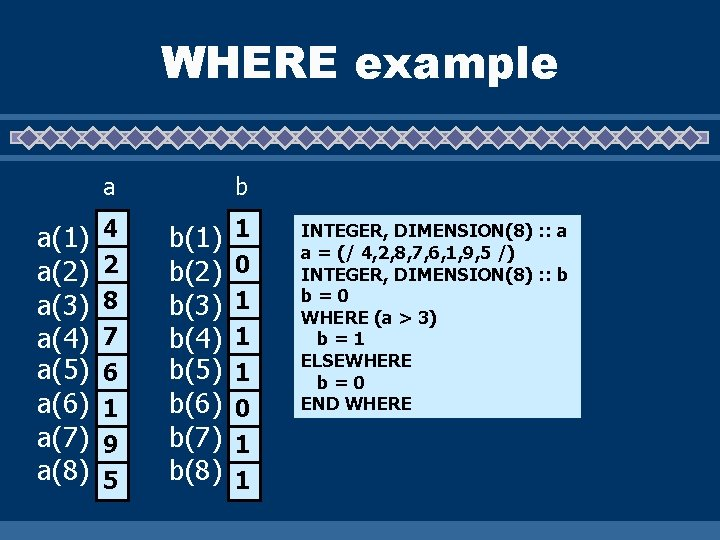WHERE example a a(1) a(2) a(3) a(4) a(5) a(6) a(7) a(8) 4 2 8 7 6 1 9 5 b b(1) b(2) b(3) b(4) b(5) b(6) b(7) b(8) 1 0 1 1 INTEGER, DIMENSION(8) : : a a = (/ 4, 2, 8, 7, 6, 1, 9, 5 /) INTEGER, DIMENSION(8) : : b b=0 WHERE (a > 3) b=1 ELSEWHERE b=0 END WHERE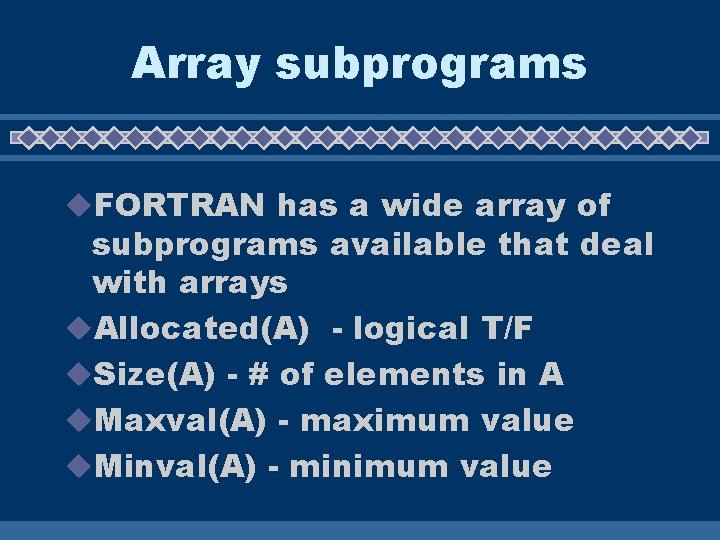Array subprograms u. FORTRAN has a wide array of subprograms available that deal with arrays u. Allocated(A) - logical T/F u. Size(A) - # of elements in A u. Maxval(A) - maximum value u. Minval(A) - minimum value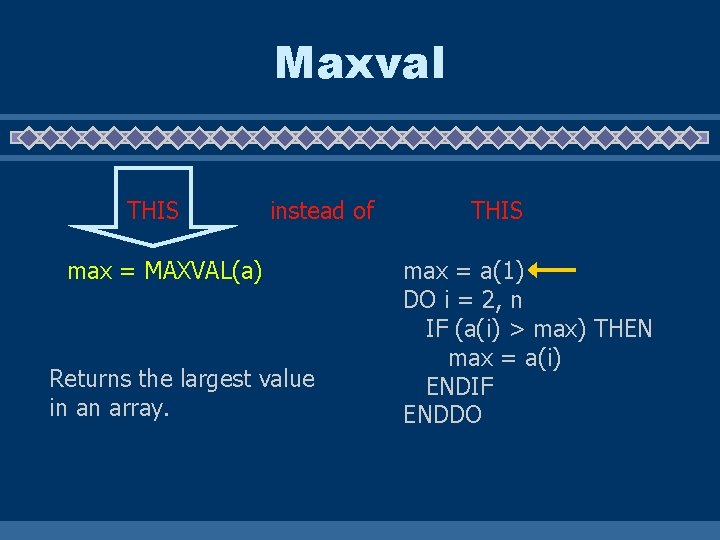Maxval THIS instead of max = MAXVAL(a) Returns the largest value in an array. THIS max = a(1) DO i = 2, n IF (a(i) > max) THEN max = a(i) ENDIF ENDDOMinval THIS instead of min = MINVAL(a) Returns the smallest value in an array. THIS min = a(1) DO i = 2, n IF (a(i) < min) THEN min = a(i) ENDIF ENDDO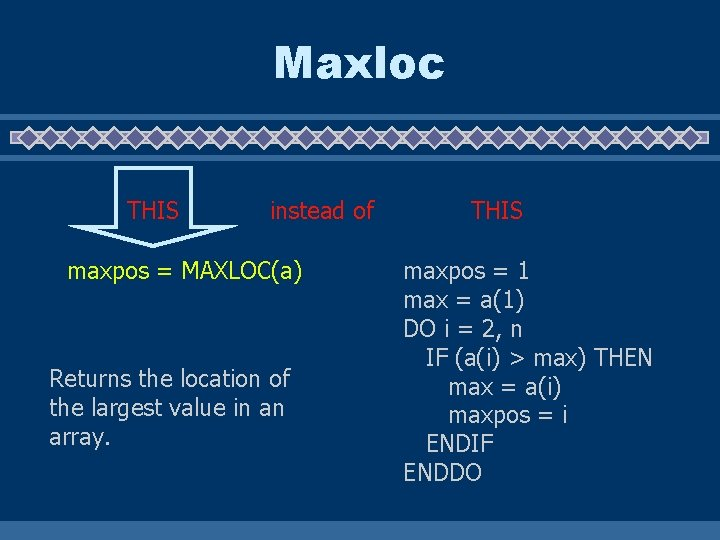Maxloc THIS instead of maxpos = MAXLOC(a) Returns the location of the largest value in an array. THIS maxpos = 1 max = a(1) DO i = 2, n IF (a(i) > max) THEN max = a(i) maxpos = i ENDIF ENDDO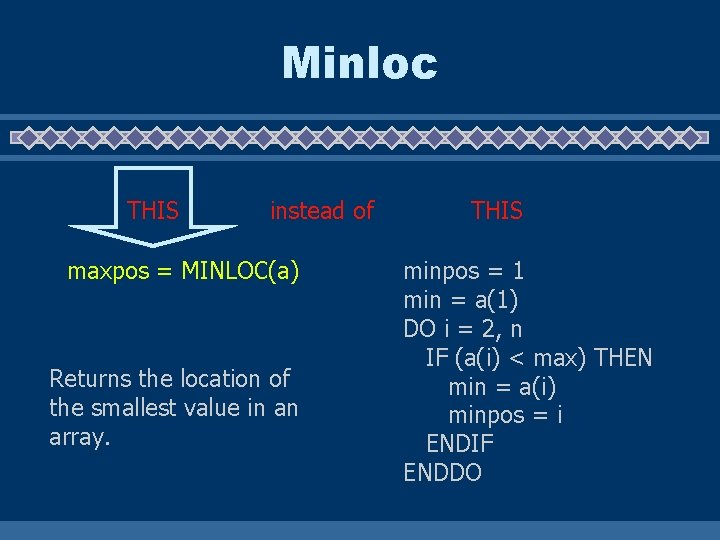Minloc THIS instead of maxpos = MINLOC(a) Returns the location of the smallest value in an array. THIS minpos = 1 min = a(1) DO i = 2, n IF (a(i) < max) THEN min = a(i) minpos = i ENDIF ENDDO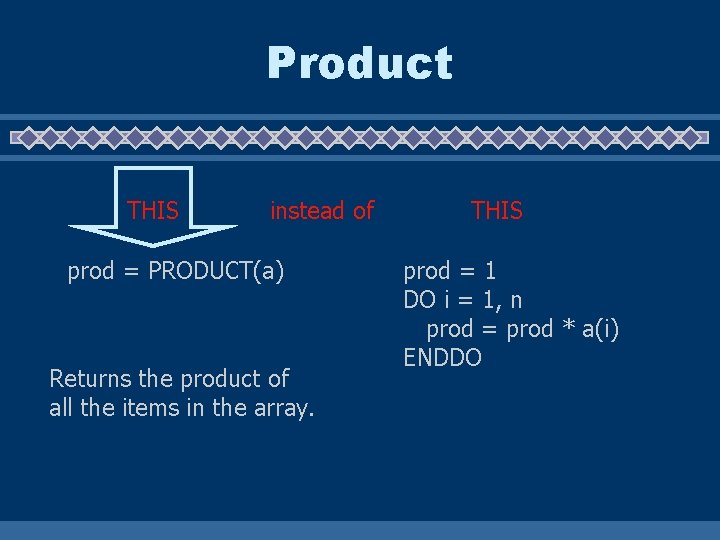Product THIS instead of prod = PRODUCT(a) Returns the product of all the items in the array. THIS prod = 1 DO i = 1, n prod = prod * a(i) ENDDO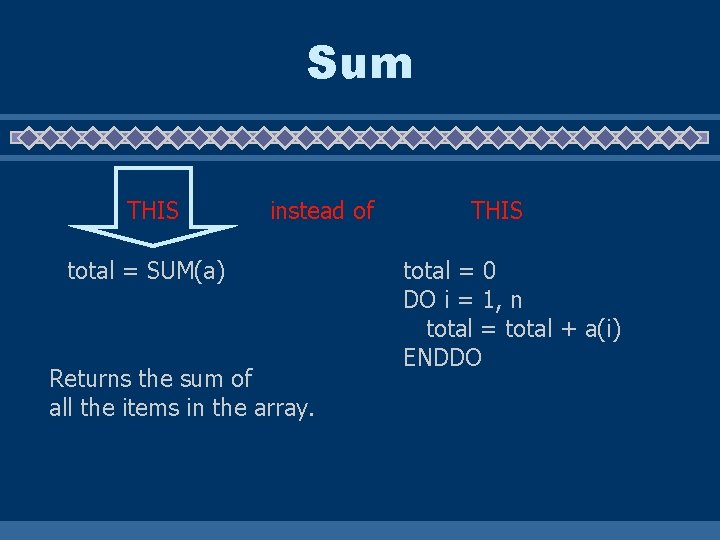Sum THIS instead of total = SUM(a) Returns the sum of all the items in the array. THIS total = 0 DO i = 1, n total = total + a(i) ENDDODot_Product THIS instead of dp = DOT_PRODUCT(a, b) Returns the dot product of all the items in the array. THIS dp = 0 DO i = 1, n dp = dp + (a(i) * b(i)) ENDDO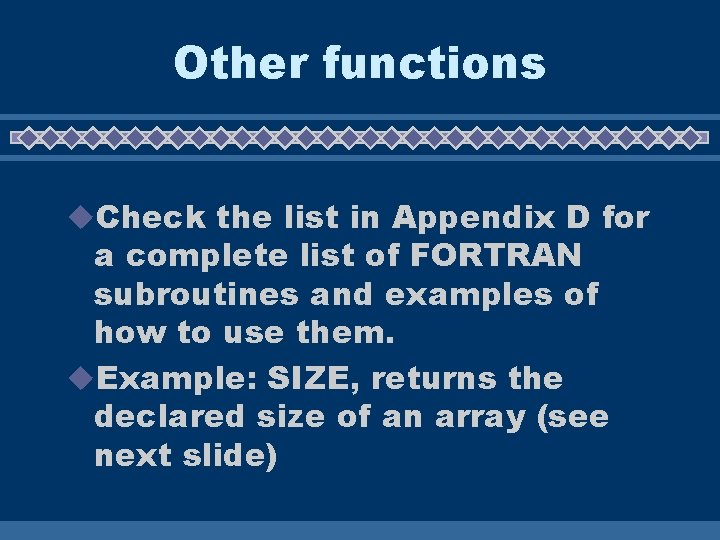Other functions u. Check the list in Appendix D for a complete list of FORTRAN subroutines and examples of how to use them. u. Example: SIZE, returns the declared size of an array (see next slide)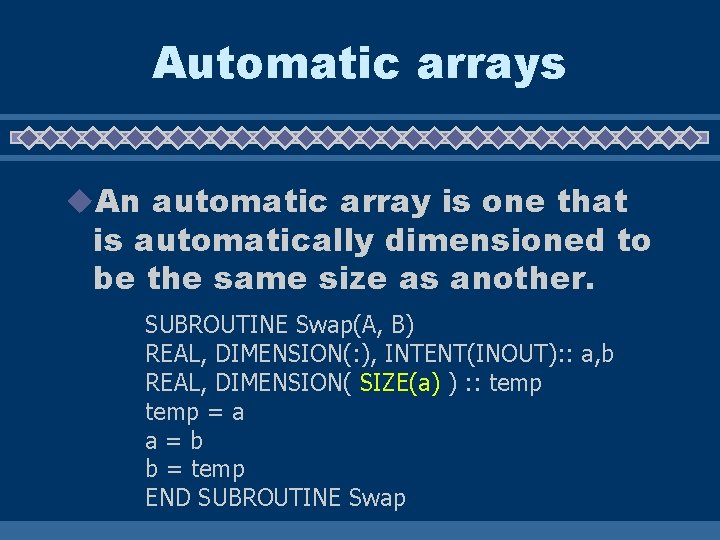Automatic arrays u. An automatic array is one that is automatically dimensioned to be the same size as another. SUBROUTINE Swap(A, B) REAL, DIMENSION(: ), INTENT(INOUT): : a, b REAL, DIMENSION( SIZE(a) ) : : temp = a a=b b = temp END SUBROUTINE Swap